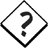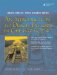# Inheritance Design

Sometimes defining an inheritance relationship helps at first (e.g., by reducing redundant code), but causes problems later when other classes must be added to the hierarchy. Some up-front analysis can help make things easier and avoid problems later.

Example 6.14, in which we derived from the abstract Shape class, demonstrates an inheritance relationship of two levels of depth. The Rectangle class was used as a classification of objects and also as a concrete class.

Is a square a kind of rectangle? Geometrically it certainly is. Here are some definitions that we borrow from elementary geometry.

• A shape is a closed two-dimensional object in the plane, with a graphical way of representing itself, together with a point that is considered its "center."
• A rectangle is a shape consisting of four straight line segments with only 90-degree angles.
• A square is a rectangle with equal sides.

As we attempt to represent an inheritance tree of classes, it helps to list the kinds of capabilities that we will need to provide for each class. They would be:

• Drawable
• Scalable
• Savable

After we describe the interface in further detail, the geometric definitions for shape classification may not lead to the ideal taxonomy for these shape classes.

As we perform an analysis, some questions arise:

• What are the common operations and features we want to describe in our abstract base classes?
• What other kinds of shapes will we use in our application?
• A rhombus is four-sided, like a rectangle, so should Rectangle derive from Rhombus?
• Should we have a base class for all four-sided objects?
• Is a Square substitutable for a Rectangle?
• Should we have a different base class for all five-sided objects?
• Should we have a general base class for polygons and represent the number of sides as an attribute?
• What are the common operations we will want to perform on all shapes?
• Is our program going to perform geometric proof searches to identify objects?
• Why do we need a Rectangle class as the base class of a Square?

Using a UML modeling tool makes it easier to try out different ideas before writing concrete code. UML diagrams are especially useful for focusing on and describing small parts of a larger system.

In Figure 6.4, we have concrete classes that serve as templates for creating the more "specific" shapes. The leaf classes are, in some cases, constrained versions of their base classes. The vector-representation, drawing, and loading/saving of the objects is handled in the abstract base classes.

Figure 6.4. Another way to represent shapesIn the geometric sense, given a circle, one can prove it is also an ellipse, because an equation exists that specifies an ellipse, with its two foci being equal. In contrast, the diagram in Figure 6.4 shows Ellipse to be a kind of Circle, with an extra point, or an extra degree of freedom. Would it make more sense to reverse the inheritance relationship? Or to have a completely different tree? Where is the is-a relationship?